#### Concept of Diffusion and Current Flow

The Diffusion Process:

The second principal method of current flow in semiconductors is diffusion. When the concentration of free charge carriers at a particular position in a semiconductor is higher than at other surrounding positions, then the atomic forces that prevail cause the carriers to migrate away from the area of higher concentration towards such of lower concentration. This procedure is termed as diffusion and gives mount to a current termed as diffusion current. This is demonstrated in figures shown below, that exhibits the changing concentration of carriers with time subsequent to a quantity of carriers has been released at a position.

Note that the movement of carriers is for all time in the direction of reducing concentration. The diffusion of carriers will carry on as long as the concentration gradient is maintained. The resultant current will flow in a direction that depends on the direction of concentration gradient and the charge on carrier.

Diffusion Current:

The rate at which diffusion occurs finds out the charge flux density due to diffusion. This mainly depends on:

dn/dx, dp/dx = carrier concentration gradients,

-q, +q = charge on the carriers,

Dn, Dp = diffusion coefficients

The diffusion coefficients are measure of relative ease with which the carriers can move in the concentration gradients. The dimensions of such coefficients are cm2s-1 (area/unit time).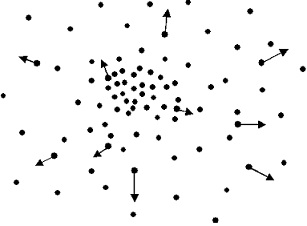Figure: The Process of Diffusion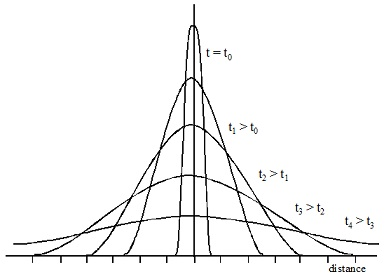Figure: Changes in Carrier Concentration with Time all through Diffusion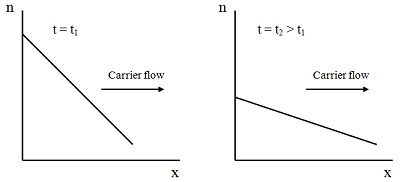Figure: Change in Concentration Gradient with Diffusion

Charge flux density for the diffusion is then:

Jn diff = -q. Dn. – (dn/dx) = q Dn (dn/dx) for electrons

Jp diff = + q. Dp. – (dp/dx) = - q Dp (dp/dx) for holes

The negative signs in front of concentration gradients above, account for the fact that carriers move in the direction of reducing concentration. Note that when the concentration gradients for both electrons and holes are in similar direction, then both types of carrier will be travelling in similar direction and the charge flows will tend to cancel one another. If, on the other hand, gradients are in opposite directions, then the flow of charge of opposite sign will tend to add altogether.

For a piece of homogeneous semi-conductor of uniform cross-sectional area A, total diffusion current due to both holes and electrons in the positive x direction is as follows:

Idiff = Jn diff A + Jp diff A = A q [Dn (dn/dx) – Dp (dp/dx)]

The polarity of gradients will find out the direction of the flow of individual carriers and, therefore, the total diffusion current.

The Einstein Relation:

The diffusion coefficient is associated to the mobility of carriers by the Einstein Relation:

Dnn = kT/q => Dn = μn (kT/q) = μn VT   for electrons

Dpp = kT/q => Dp = μp (kT/q) = μp VT   for holes

Where, VT = (kT/q) = 26mV at 300oK is termed as the thermal voltage.

Thermal Voltage is the potential difference in an electric field via which an electron with a charge of magnitude q should accelerate to gain the similar amount of energy as it has due to temperature kT.

Carrier Injection, Diffusion and Charge Continuity

Let consider a length of homogeneous p-type semi-conductor of uniform cross-sectional area A, as shown in figure below. Beneath equilibrium conditions, the concentration of majority of carriers is determined by doping concentration. When excess minority charge carriers are injected to semiconductor from an external source, then this equilibrium is disturbed as excess minority carrier’s injected start to recombine with the majority carriers present. This gives mount to modifications in the charge concentration in the material.

The rate of change of carrier concentration mainly depends on the rate of injection of surplus carriers and the rate of recombination in volume.

Notation: for electrons

Net carrier concentration = n
Equilibrium carrier concentration = n0
Excess Carrier Concentration = n’

And hence,

Excess carrier concentration is: n’ = n - n0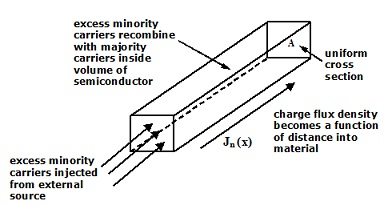Figure: Excess Charge Carriers Injected to a Semiconductor

When there is no electric field across the semiconductor and the material is uniform and homogeneous, then the injected minority carriers will move just by diffusion in x direction. The law governing this procedure is termed as the Diffusion Equation which for one dimension is as follows:

[∂n’(x)]/∂t = Dn [∂2n’(x)]/∂x2] – [n’(x)/τn]

Where tn is the average carrier lifetime before re-combination. When the supply of minority carriers in the material can be maintained by some external means and the majority carriers that recombine can as well be replaced, then this will support the recombination procedure and ultimately a steady-state condition will reached and the concentration of charge in the volume will reach a stable value and hence, [∂n’(x)]/∂t = 0

Under such conditions:

[∂2n’(x)]/∂x2 = n’(x)/Dn τn

The second order differential equation has a general solution of the form:

n’(x) = Ae-x/Ln + Be+x/Ln

As recombination can only decrease the free carrier concentration in semiconductor; there is no growth term and hence B = 0. Therefore the solution is:

n’(x) = n’(x = 0) e-x/Ln

Where n’(x = 0) is the quantity of surplus minority carriers injected to material at x = 0.

Minority carriers will penetrate a distance Ln, on average into the volume prior to recombining. This distance is equivalent to the depth of penetration that would occur if the concentrations at the boundary of material were maintained. This is related to the average carrier lifetime via the diffusion coefficient and hence:

Dn τn = Ln2

The profile of minority carrier concentration across the material to which they are injected thus has an exponential form as shown in figure below. Also note that if a thin slice of semiconductor is taken, then most of the injected carriers pass via the slice and emerge at other side however some recombined. The quantity of minority carriers that pass via the material depends on the thickness of the slice and doping concentration of the material.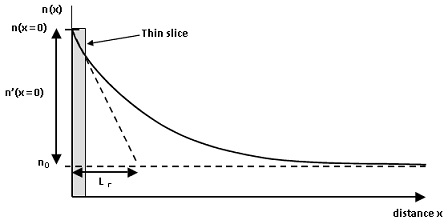Figure: Profile of Minority Carrier Concentration via Semiconductor

Latest technology based Electrical Engineering Online Tutoring Assistance

Tutors, at the www.tutorsglobe.com, take pledge to provide full satisfaction and assurance in Electrical Engineering help via online tutoring. Students are getting 100% satisfaction by online tutors across the globe. Here you can get homework help for Electrical Engineering, project ideas and tutorials. We provide email based Electrical Engineering help. You can join us to ask queries 24x7 with live, experienced and qualified online tutors specialized in Electrical Engineering. Through Online Tutoring, you would be able to complete your homework or assignments at your home. Tutors at the TutorsGlobe are committed to provide the best quality online tutoring assistance for Electrical Engineering Homework help and assignment help services. They use their experience, as they have solved thousands of the Electrical Engineering assignments, which may help you to solve your complex issues of Electrical Engineering. TutorsGlobe assure for the best quality compliance to your homework. Compromise with quality is not in our dictionary. If we feel that we are not able to provide the homework help as per the deadline or given instruction by the student, we refund the money of the student without any delay.